Next: Aberration, the Fizeau experiment Previous: Criticism of the relativistic experiments   Contents

## The Michelson-Morley experiment

Light is known to behave in various phenomena as either a particle or a wave (the statement about corpuscular-wave dualism bears no relation to the subject under consideration). At first, let us suppose light to possess a corpuscular nature. Then the Michelson - Morley interferometer may be represented as two mutually perpendicular arms with a single ideal reflector at the middle and two reflectors at the ends of the arms (Fig. 3.1).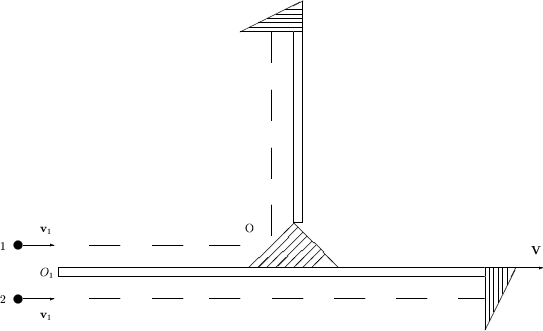Let the two particles moving parallel to each other at velocity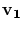(relative to the "universal reference system") fall into the given set-up, which, in its turn moves at velocity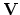with speed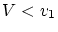(relative to the same reference system). Then at point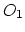the speed of particles relative to the set-up will be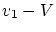. After reflection at the set-up center, particle 1 will move in the perpendicular direction at the same speedrelative to the set-up. The particles will reflect from the ends of arms simultaneously. Likewise, they will reach simultaneously both point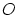and point. No difference in speeds of these two particles for two mutually perpendicular directions will be observed, regardless of velocitiesand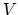. Thus, if the light is supposed to be a flow of particles, then the experiments by Michelson - Morley (Kennedy - Thorndike, Tomachek, Bonch-Bruevich and Molchanov et al.) could not give any positive result.

Let us now suppose light possesses a wave nature. In this case, light speed can only depend on the properties of the propagation medium (ether or vacuum) and/or intrinsic characteristics of the propagating light itself. If we accept a hypothesis that ether exists, then light speed depends on the properties of this medium (by analogy to sound). It is obvious then that light velocity cannot be added with source velocity (the boom from a supersonic aircraft propagates at the velocity fixed by the medium, and, as a result, the aircraft outstrips the sound). It is also obvious that, since light interacts with both matter (it scatters or absorbs) and ether (it propagates), then some interaction between ether and matter should also be observed. But in the Michelson - Morley experiment, something improbable was assumed; namely, a rigid "binding" of light to ether, along with absolutely no interaction of ether with bodies, (i.e. no ether entrainment by the Earth or by the interferometer). Of course, the theory would be complicated in the case of partial entrainment of the ether (and for some local experiments an ether entrainment can practically be complete inside the narrow boundary layer). However, this fact in no way disproves the ether hypothesis (but relativists, like a drunkard under a street lamp, call to seek not there where it can be really found, but simply there where it were easily to look). We shall briefly discuss the ether concept below, but now we shall rest on the classical relativity principle (in vacuum), since for all paradoxes of the SRT and corollaries of this book it is not important whether we have vacuum or ether.

If light is a wave, then only the light frequency changes with source velocity. So, for given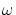, the light speed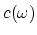does not depend on the source velocity. Here we have in mind the following situation: the light waves of the same frequency are identical to each other; and if we perceive the light of frequency, then it does not matter, whether it was emitted by a source at the same frequency, or if it was emitted at another frequency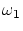, and due to source motion the frequency changed: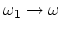(the Doppler effect). In both cases, the measured value ofis the same.

Now we return to the Michelson - Morley experiment and similar ones by other researchers. Since the incident light, the light passed through the thin plate, and the light reflected from the mirrors, all have the same frequency in the same observation system, the light speedremained constant for the two mutually perpendicular directions, and the experiments could not detect anything. Tauson's experiment with two similar lasers could not discover anything either, because in converging the beams to a single pattern (in the same direction), the frequencies become equal, and no regular beatings are observed. Thus, the attempt to find changes in light speed from the experiments with the same fixed frequency is wrong in itself. The only dependence we may try to discover is: all other dependences can enter only indirectly, through the Doppler effect.

For methodical purposes we shall consider some apparent errors from textbooks. When researchers proceed from the "classical view-point" (i.e. the hypothesis of motionless, non-involved ether), they often calculate the difference in times of beams propagation in an interferometer using a strange scheme , in which the reflection law does not "work": the angle of reflection does not equal the angle of incidence (Fig. 3.2).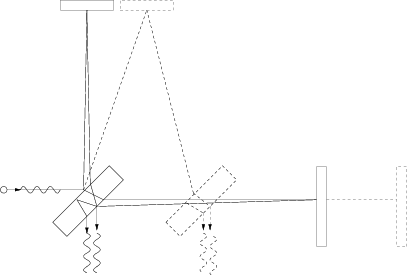This "fact" contradicts experiments. In such a circumstance, it is at minimum necessary to explain the mechanism of such a deviation, and to determine its effect on the experiment (it could be made in the assumption of classical laws for the addition of light velocity and the velocity of an interferometer's mirror). It is not clear either how we can guess the angle ensuring the interference of the same beam. Actually, since all data are registered only by the observer moving together with the interferometer, the experiment must be analyzed only from the viewpoint of this observer as well .

Time synchronization according to Einstein's method introduces artificial limitations even into the ideas of experiments. Obviously, by virtue of the reversibility of relative motion (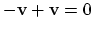), only an odd-powers effect can exist for the velocity dependence of light speed. However, the Michelson-Morley experiments, and some other ones, try to determine light speed as a mean velocity for two mutually opposite directions (for a closed path). Therefore, a single classical linear dependence on the velocity of motion of a system is mutually excluded. Thus, any similar approach already substitutes the postulate of constancy of light speed, which should be verified experimentally.

The Michelson-Morley experiment and its analogs do not contradict the Galileo principle and have been considered in detail from the empty space viewpoint above. We consider now the original idea of the experiment from the viewpoint of ether concepts. Note that the Fresnel entrainment coefficient can always be slightly corrected in such a manner, that the experiments of both 1-st and 2-nd order be confirmed to a practical accuracy. For the sake of justice one should note that the Michelson experiment and its analogs (in spite of the disputes concerning the instrument structure and the theory) have always confidently, with allowance for possible errors, given a nonzero velocity of the ether wind [94,95]. Marinov [90,91] and Silvertooth  have found a correct velocity relative to a relic radiation. Only at instrument screening with a metal casing the result occurred to be close to zero one. Not accepting the ether theory unconditionally, nevertheless, we recall for the sake of objectivity, that all instruments are vacuumed now (i.e. made a locally closed system). And, for example, the local speed of sound in airplane's saloon will remain constant (independent on the wind outside) even at supersonic motion of an airplane. The ether point of view does not contradict the obtained results: Fresnel's entrainment for metal bodies is complete (Hertz's electrodynamics is valid for metals), and, hence, the ether is resting locally inside the metal casing relative to an instrument, and searching for the ether wind inside is senseless. Yet another moment is usually hidden by relativists. Even in the absence of metal casing, the presence of a thin glass plate (or air in the original experiments) leads to light reradiation from these local rest elements. As the result, in the ether concept the really measured velocity must be wittingly less than the velocity of orbital motion of the Earth. Thus, the Michelson - Morley experiment does not witness the light speed constancy and does not testify against any classical principles.Next: Aberration, the Fizeau experiment Previous: Criticism of the relativistic experiments   Contents
Sergey N. Arteha